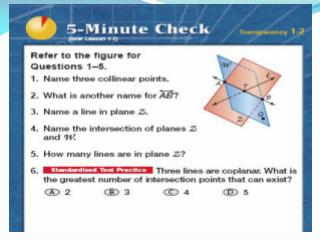DownloadDownload PresentationLine Segments

# Line Segments

Télécharger la présentation## Line Segments

- - - - - - - - - - - - - - - - - - - - - - - - - - - E N D - - - - - - - - - - - - - - - - - - - - - - - - - - -
##### Presentation Transcript

1. Line Segments Section 1.3 Sol:G.4c

2. Line Segment • Is a part of a line that consists of two points, called endpoints, and all the points between. • Symbolized by: • Distance: The distance between the points A and B written as AB is the absolute value of the difference of the coordinates of x1 and x2. Denoted: AB = |x2 – x1| Name of Points A B x1 x2 Coordinates

3. Ex: Find the Distance

4. Segment addition Postulate • If B is between A and C, then AB + BC = AC. • If AB + BC = AC, Then B is between A and C. A B C

5. Example 99 miles Bismarck Dickenson Fargo 289 miles The cities shown on the map lie approximately in a straight line. Use the given distances to find the distance from Bismarck to Fargo.

6. Try These:1. 380 miles 360 miles Lubbick Tulsa St. Louis What is the distance from Lubbick to St. Louis?

7. 36 F G H 2. Use the diagram to Find GH 3. Find y, then find PQ and QR. 21 3y+1 Q P R 2y 21

8. Try These: Solve for x, then find UT and TS1. 5x - 3 7x U T S 45

9. Congruent Segments • Line segments that have the same length / measure. • Ex: A B D C AB= CD Equal AB CD Congruent

10. Example: Find X and ST if S is between R and T • RS = 12, ST = 2x, RT = 34 • RS = 16, ST = 2x, RT = 5x + 10

11. Assignments Classwork: Workbook Pg 11-12 1-20 even 27,28. Homework: Pg 23-25 1-5, 7,12,14,16,19,20,27,37,38,39